How to Sum Top or Bottom N Values with Criteria in Excel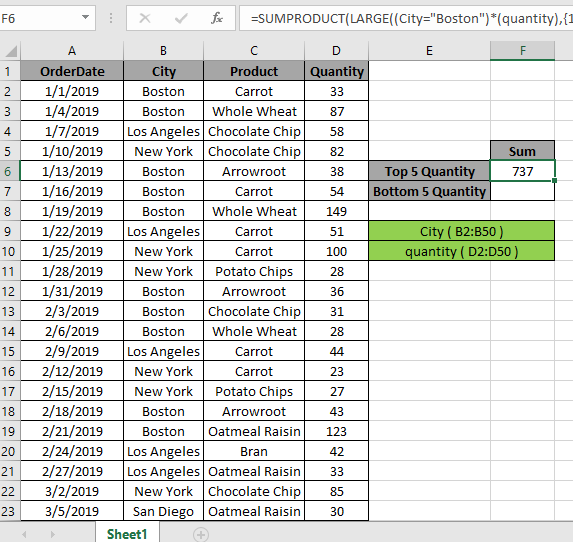In the previous article we learned how to sum up top or bottom N values. In this article we try to sum up top or bottom N values with a criteria.

Sum of TOP N values with criteria

How to solve the problem?

For this article we will be required to use the SUMPRODUCT function. Now we will make a formula out of these functions. Here we are given a range and a criteria. We need to get the top 5 values in the range and get the sum of the values based on the given criteria.

Generic formula:

= SUMPRODUCT ( LARGE ( (list = criteria) * (range), { 1 , 2 , .... ,n } } )

list: criteria list

Criteria: criteria to match

range: range of values

values: numbers separated using the commas like if you wish to find the top 3 values, use { 1 , 2 , 3 }.

Example:

Here we have the dataset values from A1:D50.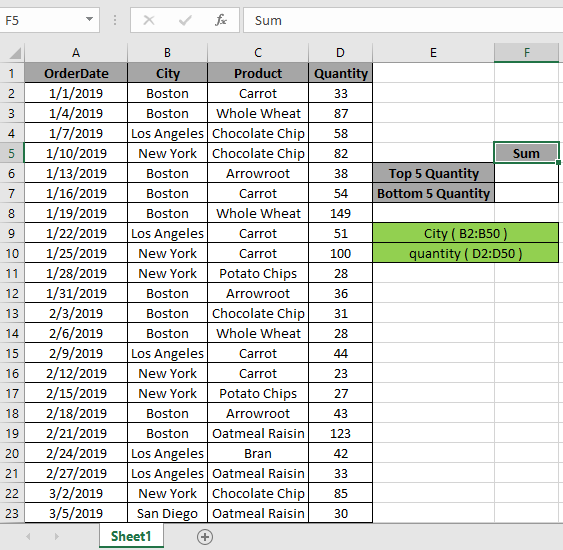Firstly, we need to find the top five values using the LARGE function which matches the city "Boston" and then sum operation be performed over those 5 values. Now we will use the following formula to get the sum

Use the Formula:

= SUMPRODUCT ( LARGE ( ( City = "Boston" ) * (quantity) , { 1 , 2 , 3 , 4 , 5 } ) )

Explanation:

• City's "Boston" matches the City range mentioned. This returns an array of true and false.
• The LARGE function returns the top 5 numerical values from the quantity range and returns the array to the SUMPRODUCT function.

= SUMPRODUCT { 193 , 149 , 138 , 134 , 123 }

• SUMPRODUCT function gets an array of top 5 values, with an array of top 5 numbers returning the SUM of those numbers.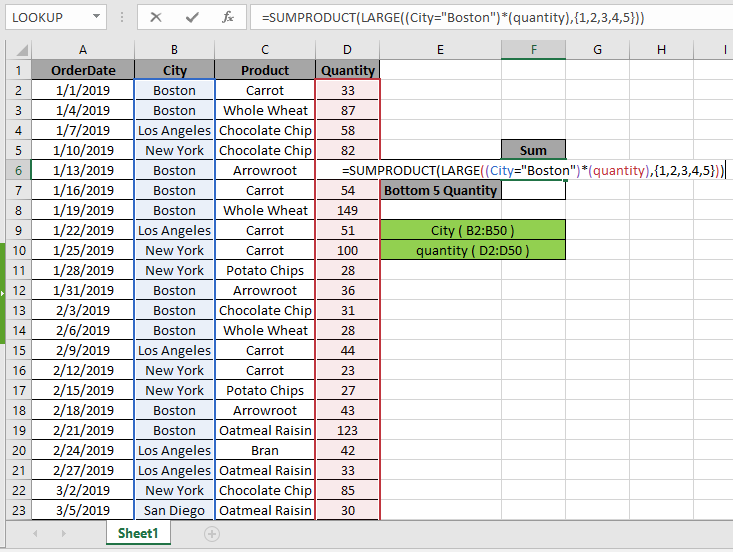Here the City & quantity range is given as the named range. Press Enter to get the SUM of the top 5 numbers.As you can see in the above snapshot, that sum is 737. The sum of the values 193 + 149 + 138 + 134 + 123 = 737.

You can check the above values in dataset using the excel filter option. Apply the filter to the City & quantity header and click the arrow button on the city header which appears. Follow the steps as shown below.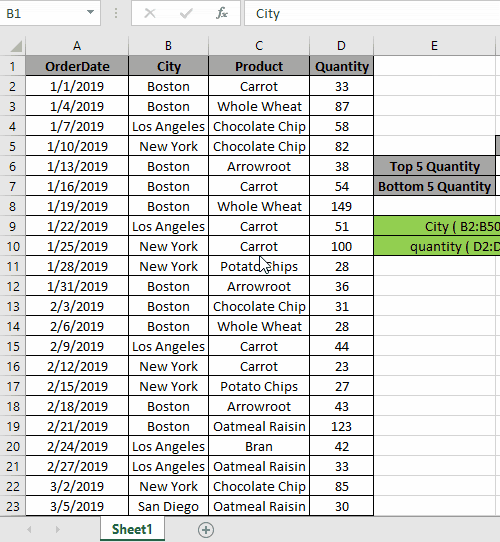Steps:

1. Select the City header cell. Apply filter using shortcut Ctrl + Shift + L
2. Click the arrow which appears as a filter option.
3. Select the (Select All) option.
4. Select only the city of Boston.
5. Select the quantity header now.
6. Sort the list from largest to smallest and you can view all the top 5 values which we calculated using the formula.

As you can see in the above gif all the 5 values which match the given criteria. This also means the formula works fine to get the count of these values

LARGE N numbers

The above process is used to calculate the sum of a few numbers from the top. But to calculate for n (large) number of values in a long range.

Use the formula:

= SUMPRODUCT ( LARGE ( ( City = "Boston" ) * (quantity), ROW ( INDIRECT ( "1:10" ) )

Here we generate sum of top 10 values via getting an array of 1 to 10 { 1 ; 2 ; 3 ; 4 ; 5 ; 6 ; 7 ; 8 ; 9 ; 10 } using the ROW & INDIRECT Excel functions.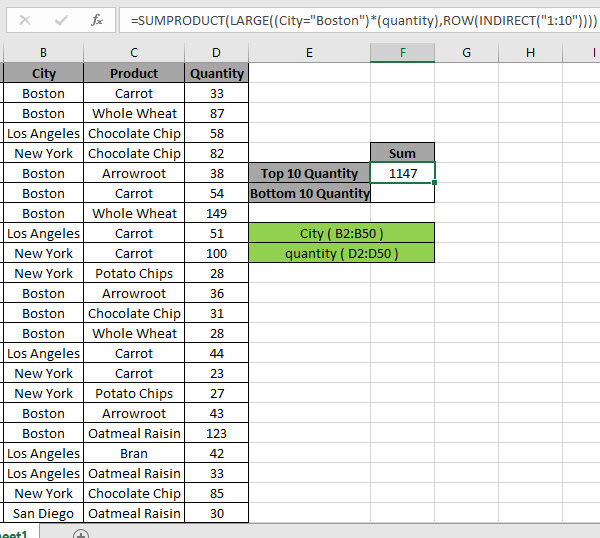Here we have the sum of the top 10 numbers which results in 1147.

Sum of Bottom N values with criteria

How to solve the problem?

For this article we will be required to use the SUMPRODUCT function. Now we will make a formula out of these functions. Here we are given a range and we need to bottom 5 values in range and get the sum of the values.

Generic formula:

{ = SUM ( SMALL ( IF ( City = "Boston" , quantity ) , { 1 , 2 , 3 , 4 , 5 } ) ) }

Range: range of values

Values : numbers separated using the commas like if you wish to find the bottom 3 values, use { 1 , 2 , 3 }.

Example:

All of these might be confusing to understand. So, let's test this formula via running it on the example shown below.

Here we have a range of values from A1:D50.Here the City & quantity range is given as using the named range excel tool.

Firstly, we need to find the bottom five values using the SMALL function which matches criteria and then sum operation be performed over those 5 values. Now we will use the following formula to get the sum
Use the Formula:

{ = SUM ( SMALL ( IF ( City = "Boston" , quantity ) , { 1 , 2 , 3 , 4 , 5 } ) ) }

DON'T use curly braces manually. Curly braces applied using the Ctrl + Shift + Enter in place of just Enter.

Explanation:

• SMALL function with IF function returns the bottom 5 numerical values which matches City "Boston" and returns the array to the SUM function.

= SUM ( { 23 , 27 , 28 , 28 , 30 } ) )

• SUM function gets the array of bottom 5 values, which has an array of bottom 5 numbers returns the SUM of those numbers used with CTRL +SHIFT + ENTER.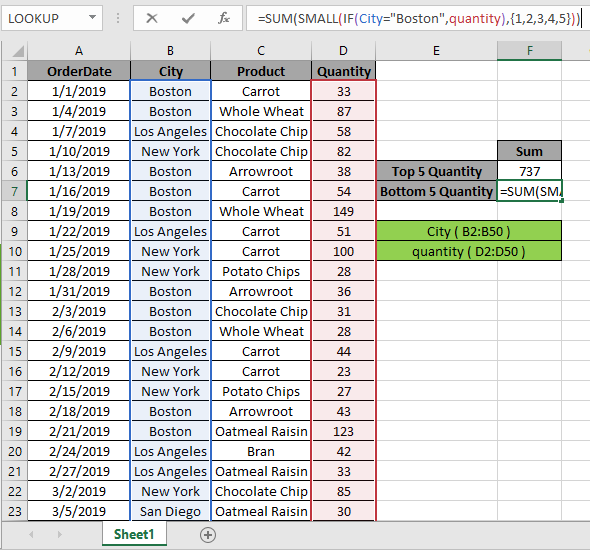Here the City & quantity range is given as the named range. Press Ctrl + Shift + Enter to get the SUM of the bottom 5 numbers as this is an array formula.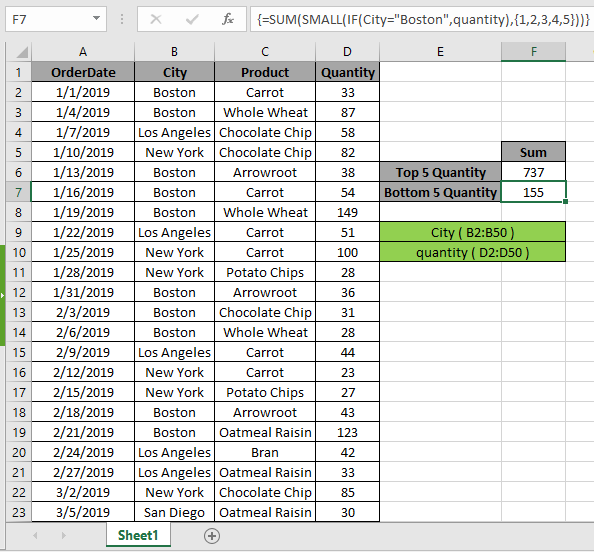As you can see in the above snapshot that sum is 136.

The above process is used to calculate the sum of a few numbers from the bottom. But to calculate for n (large) number of values in a long range.

Use the formula:

{ = SUM ( SMALL ( IF ( City = "Boston" , quantity ) , ROW ( INDIRECT ("1:10") ) ) ) }

DONOT use the curly brackets manually. Use the Ctrl + Shift + Enter in place of using the Enter.
Here we generate sum of bottom 10 values via getting an array of 1 to 10 { 1 ; 2 ; 3 ; 4 ; 5 ; 6 ; 7 ; 8 ; 9 ; 10 } using the ROW & INDIRECT Excel functions.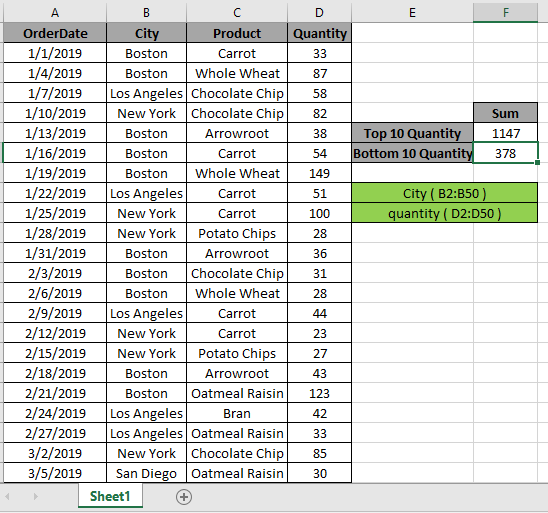Here we have the sum of the bottom 10 numbers which will result in 155.

Here are some observational notes shown below.

Notes:

1. The formula only works with numbers.
2. The formula only works when there are no duplicates on the lookup table
3. The SUMPRODUCT function considers non - numeric values ( like text abc ) and error values ( like #NUM! , #NULL! )  as null values.
4. The SUMPRODUCT function considers logic value TRUE as 1 and False as 0.
5. The argument array must be of the same length as the function.

Hope this article about how to Return Sum of top 5 values or bottom 5 values with criteria in Excel is explanatory. Find more articles on SUMPRODUCT functions here. Please share your query below in the comment box. We will assist you.

If you liked our blogs, share it with your friends on Facebook. And also you can follow us on Twitter and Facebook. We would love to hear from you, do let us know how we can improve, complement or innovate our work and make it better for you. Write us at info@exceltip.com

Related Articles

How to use the SUMPRODUCT function in Excel: Returns the SUM after multiplication of values in multiple arrays in excel.

SUM if date is between : Returns the SUM of values between given dates or period in excel.

Sum if date is greater than given date: Returns the SUM of values after the given date or period in excel.

2 Ways to Sum by Month in Excel: Returns the SUM of values within a given specific month in excel.

How to Sum Multiple Columns with Condition: Returns the SUM of values across multiple columns having condition in excel

How to use wildcards in excel : Count cells matching phrases using the wildcards in excel

Popular Articles

50 Excel Shortcut to Increase Your Productivity

Edit a dropdown list

Absolute reference in Excel

If with conditional formatting

If with wildcards

Vlookup by date

Convert Inches To Feet and Inches in Excel 2016

Join first and last name in excel

Count cells which match either A or B

Terms and Conditions of use

The applications/code on this site are distributed as is and without warranties or liability. In no event shall the owner of the copyrights, or the authors of the applications/code be liable for any loss of profit, any problems or any damage resulting from the use or evaluation of the applications/code.